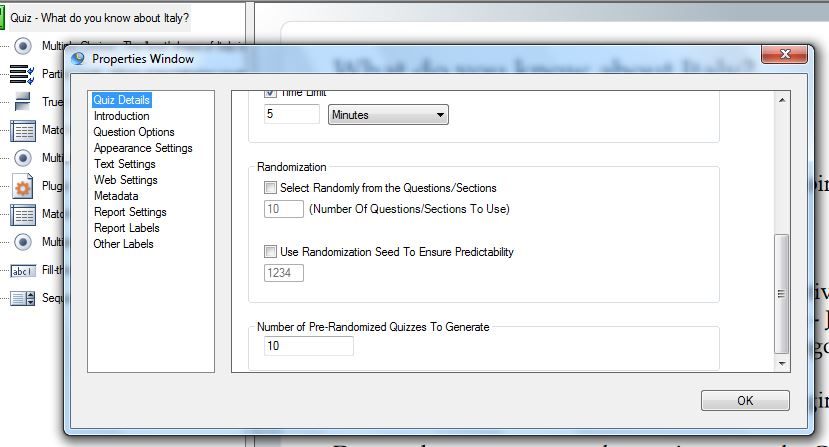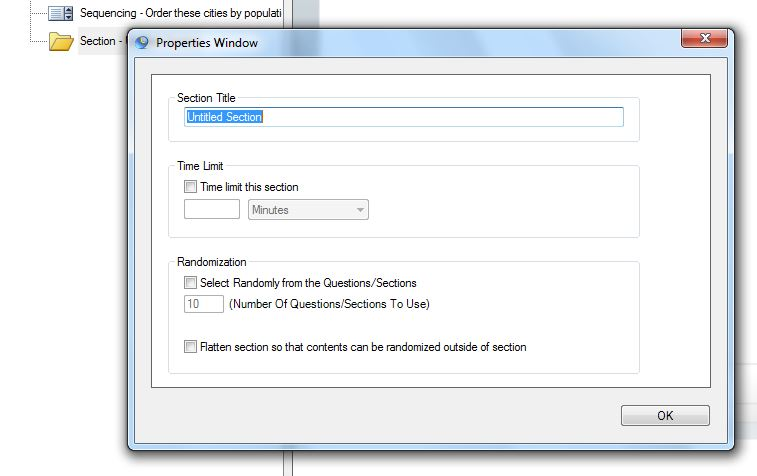# How do I create a quiz with random questions?

You can use random questions if you want users to retake a quiz repeatedly and to find out if a learner really knows his stuff or has just memorized the answer sequence from previous attempts.

It works best if your quiz has a large bank of questions because it lessens the likelihood of them seeing the same questions over and over. To make a random test, here are the steps:Open the quiz properties window by right clicking while your mouse is over the green quiz icon and selecting Properties.

In the quiz properties window, go to the Randomization section. Tick the box Select Randomly from the Questions/Sections and add the number of random questions you want in the field below. For example, you can choose to give 10 random questions out of 100 questions.

As part of the publishing process Question Writer generates a number of quizzes with 10 questions randomly selected from your pool of 100.  You need to specify how many pre-generated quizzes it publishes in the box Number of Pre-Randomized Quizzes to Generate.  The higher the number the less likely people retaking the quiz will be to find the same quiz twice.

When you add sections to your quiz you can choose to randomize what appears from each section.  For example if you want a random quiz but want to be sure that there are a certain number of questions from each subject.  Firstly add the sections and add questions to each section.  You can then open the section properties window and set up the randomization options.If you want to use sections for admin reasons but not separate how the questions appear in the quiz then tick the Flatten Section so that….  box in the section Properties.  Here is a link to a short video tutorial that describes how to use randomization, sections and section flattening.

If you add sections and then use the Quiz Properties Randomization then you will be opting to randomly select a number of sections to include in the quiz….so if you want all sections to appear you just need to add the number of sections that you have created.

## Randomization Seed

The Randomization feature allows you to have produce a hard copy of your quiz that you can be sure will reflect what your users are seeing.   You can put any number in the box within the range of the number of random quizzes that you will pre-generate.  Eg if you are going to pre-generate 10 quizzes you can put any number between 1 and 10 and change it if you want to see a different quiz.  The consistent randomization is part of the quiz properties so it operates over the quiz as a whole.